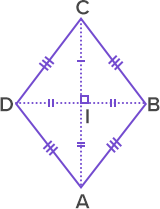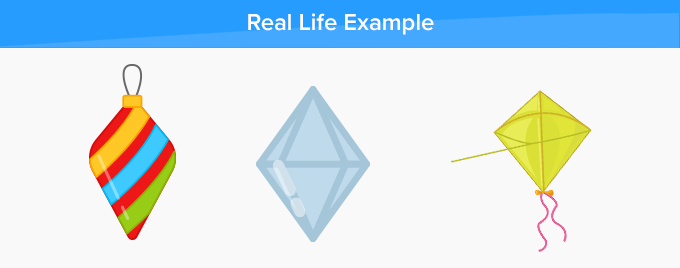# Rhombus - Definition with Examples

The Complete K-5 Math Learning Program Built for Your Child

• 40 Million Kids

Loved by kids and parent worldwide

• 50,000 Schools

Trusted by teachers across schools

• Comprehensive Curriculum

Aligned to Common Core

What is Rhombus?

Rhombus is a special type of a parallelogram whose all sides are equal.

The difference between a square and rhombus is that all angles of a square are right angles, but the angles of a rhombus need not be right angles.

So, a rhombus with right angles becomes a square.

We can say, “Every square is rhombus but all rhombus are not square.

Properties of a rhombus

The given table describes the properties of a rhombus.All sides of a rhombus are equal.  Here, AB = BC = CD = DA.Diagonals bisect each other at 90°  Here, diagonals AC and BD bisect each other at 90°Opposite sides are parallel in a rhombus.  Opposite angles are equal in a rhombus.  Here, AB ∥ CD and AD ∥ BC.  ∟A = ∟C and ∟B = ∟D.Adjacent angles add up to 180°.  ∠A + ∠B = 180°  ∠B + ∠C = 180°  ∠C + ∠D = 180°  ∠A + ∠D = 180°

Real-life examples

Rhombus can be found in a variety of things around us, such as a kite, windows of a car, rhombus-shaped earring, the structure of a building, mirrors, and even a section of the baseball field.Fun Facts about rhombus The term ‘rhombus’ has been derived from the Greek word ‘rhombos (ῥόμβος)’ which means something that spins, and this term was eventually derived from the Greek verb ‘rhembō (ῥέμβω)’ which means to turn round and round.

Won Numerous Awards & Honors